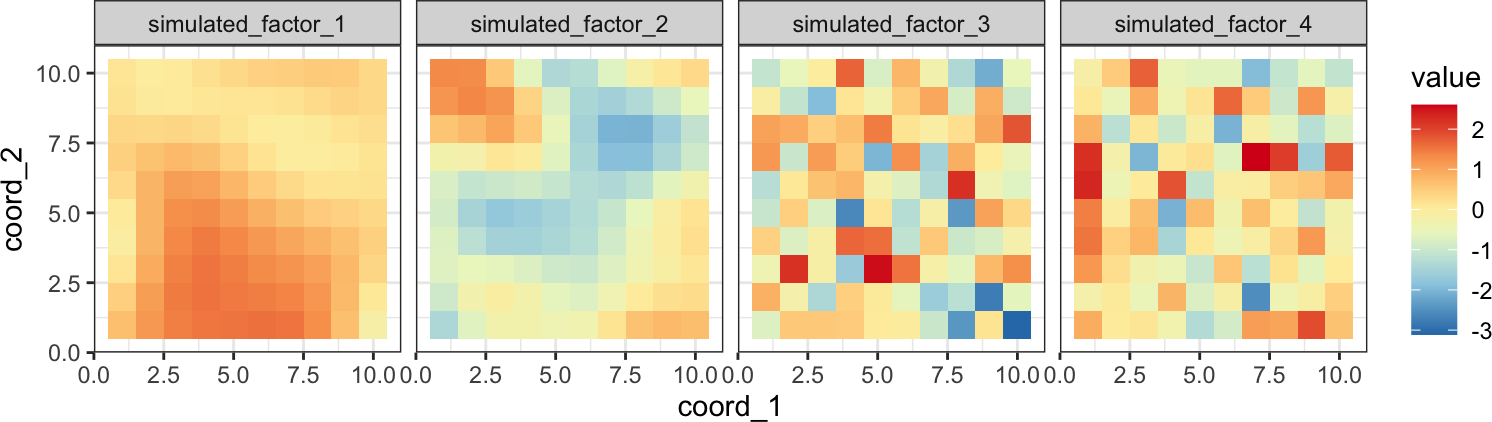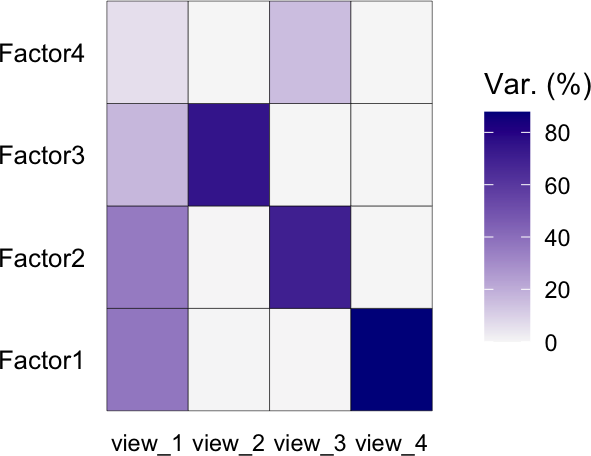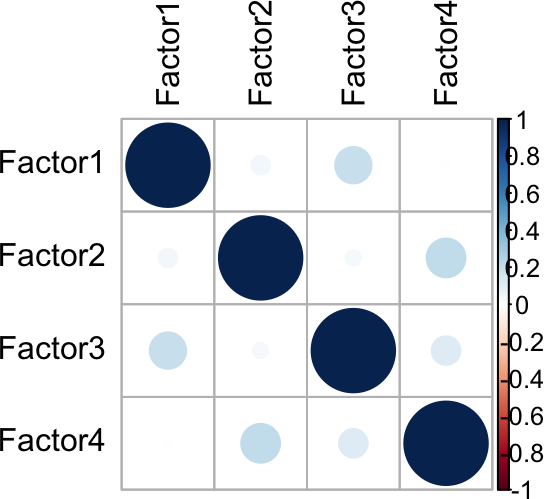``````library(MOFA2)
library(tidyverse)
library(pheatmap)
library(RColorBrewer)``````

# 1 Spatial data: Simulate an example data set

To illustrate the MEFISTO method in MOFA2 we simulate a small example data set with 4 different views and two covariates defining a spatial grid using `make_example_data`. The simulation is based on 4 factors, two of which vary smoothly along the spatial coordinates (with different lengthscales) and two are independent of space.

``````set.seed(2020)

# generate spatial grid and set number samples
sample_cov <- expand_grid(x = 1:10, y =1:10) %>% t()
N <- ncol(sample_cov)

# generate example data
dd <- make_example_data(sample_cov = sample_cov, n_samples = N,
n_factors = 4, n_features = 200,
n_views = 4, lscales = c(3, 2, 0, 0))

# input data
data <- dd\$data

# covariate matrix with samples in columns and
# spatial coordinates in rows
coords <- dd\$sample_cov
rownames(coords) <- c("coord_1", "coord_2")``````

Let’s have a look at the simulated latent processes, which we want to recover:

``````df <- data.frame(dd\$Z, t(coords))
df <- gather(df, key = "factor", value = "value", starts_with("simulated_factor"))
ggplot(df, aes(x=coord_1, y=coord_2, fill = value)) +
geom_tile() +
facet_grid( ~ factor) +
theme_bw() +# 2 MEFISTO framework

Using the MEFISTO framework is very similar to using MOFA2. In addition to the omics data, however, we now additionally specify the spatial positions for each sample. If you are not familiar with the MOFA2 framework, it might be helpful to have a look at MOFA2 tutorials first.

## 2.1 Create a MOFA object with covariates

To create the MOFA object we need to specify the training data and the covariates for pattern detection and inference of smooth factors. Here, `sample_cov` is a matrix with samples in columns and spatial coordinates in rows. The sample order must match the order in data columns. Alternatively, a data frame can be provided containing one `sample` columns with samples names matching the sample names in the data.

First, we start by creating a standard MOFA model.

``sm <- create_mofa(data = data)``
``## Creating MOFA object from a list of matrices (features as rows, sample as columns)...``

Now, we can add the spatial covariates, that we want to use for training.

``````sm <- set_covariates(sm, covariates = coords)
sm``````
``````## Untrained MEFISTO model with the following characteristics:
##  Number of views: 4
##  Views names: view_1 view_2 view_3 view_4
##  Number of features (per view): 200 200 200 200
##  Number of groups: 1
##  Groups names: group1
##  Number of samples (per group): 100
##  Number of covariates per sample: 2
## ``````

We now successfully created a MOFA object that contains 4 views, 1 group and 2 covariates giving the spatial coordinates.

## 2.2 Prepare a MOFA object

Before training, we can specify various options for the model, the training and the data preprocessing. If no options are specified, the model will use the default options. See also `get_default_data_options`, `get_default_model_options` and `get_default_training_options` to have a look at the defaults and change them where required. For illustration pruposes, we only use a small number of training iterations.

Importantly, to activate the use of the covariate for a functional decomposition (MEFISTO) we now need to specify `mefisto_options` in addition to the standard MOFA options. For this you can just use the default options (`get_default_mefisto_options`), unless you want to make use of advanced options such as alignment across groups.

``````data_opts <- get_default_data_options(sm)

model_opts <- get_default_model_options(sm)
model_opts\$num_factors <- 4

train_opts <- get_default_training_options(sm)
train_opts\$maxiter <- 100

mefisto_opts <- get_default_mefisto_options(sm)

sm <- prepare_mofa(sm, model_options = model_opts,
mefisto_options = mefisto_opts,
training_options = train_opts,
data_options = data_opts)``````

## 2.3 Run MOFA

Now, the MOFA object is ready for training. Using `run_mofa` we can fit the model, which is saved in the file specified as `outfile`. If none is specified, the output is only saved in a temporary location.

``sm <- run_mofa(sm)``

## 2.4 Down-stream analysis

### 2.4.1 Variance explained per factor

Using `plot_variance_explained` we can explore which factor is active in which view. `plot_factor_cor` shows us whether the factors are correlated.

``plot_variance_explained(sm)````r <- plot_factor_cor(sm)``### 2.4.2 Plot the factors on the covariate

The MOFA model has learnt scale parameters for each factor, which give us an indication of the smoothness per factor along the covariate (here space) and are between 0 and 1. A scale of 0 means that the factor captures variation independent of space, a value close to 1 tells us that this factor varys very smoothly along space.

``get_scales(sm)``
``````##     Factor1     Factor2     Factor3     Factor4
## 0.001815212 0.196601165 0.999358744 0.997614847``````

In this example, we find two factors that are non-smooth and two smooth factors. Using `plot_factors_on_cov_2d` we can plot the factors along the spatial coordinates, where we can distinguish smooth and non smooth variation across space.

``plot_factors_vs_cov(sm) ``